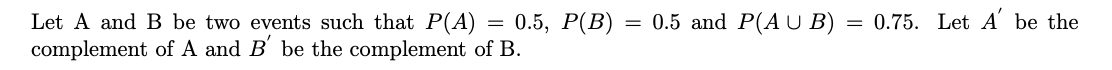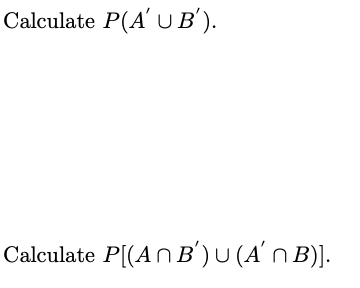Home / Expert Answers / Statistics and Probability / let-mathrm-a-and-mathrm-b-be-two-events-such-that-p-a-0-5-p-b-0-5-and-pa573

# (Solved): Let $$\mathrm{A}$$ and $$\mathrm{B}$$ be two events such that $$P(A)=0.5, P(B)=0.5$$ and $$...Let \( \mathrm{A}$$ and $$\mathrm{B}$$ be two events such that $$P(A)=0.5, P(B)=0.5$$ and $$P(A \cup B)=0.75$$. Let $$A^{\prime}$$ be the complement of $$\mathrm{A}$$ and $$B^{\prime}$$ be the complement of $$\mathrm{B}$$. Calculate $$P\left(A^{\prime} \cup B^{\prime}\right)$$ Calculate $$P\left[\left(A \cap B^{\prime}\right) \cup\left(A^{\prime} \cap B\right)\right]$$.

We have an Answer from Expert